# 小白学Java 第10课：if 条件语句

【相关链接】

【1】理解 if 条件语句

if 在英文中是一个单词，这个单词相信大家只要会一丢丢英文就知道他的意思，没错，它就是 "如果" 的意思。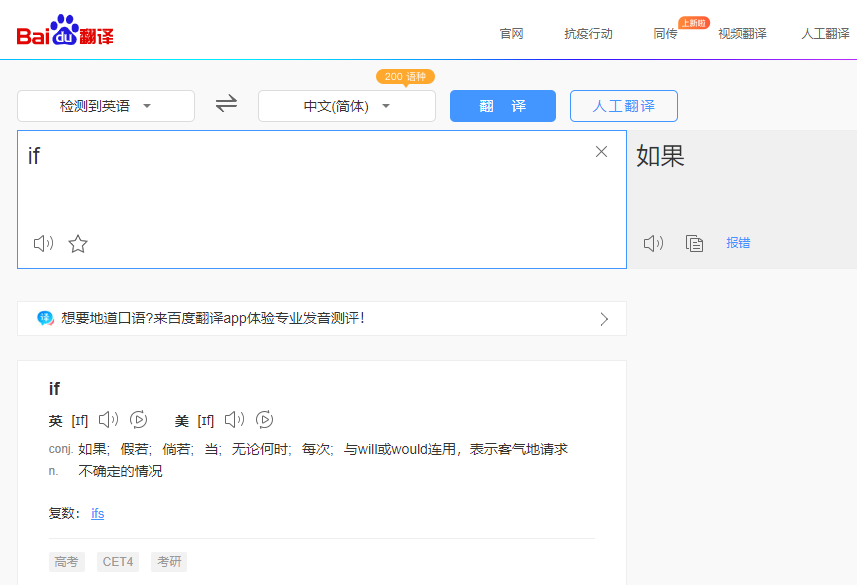if(明天不下雨) {
我就出去玩
} else {
我就呆在家里
}

【2】if ... else ...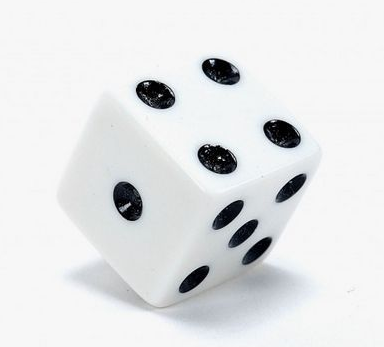if(点数 <=3 ) {
小
} else {
大
}

if(点数 > 3 ) {
大
} else {
小
}

package com.java;

/**
* if 条件语句
*/
public class Test10 {

public static void main(String[] args) {
int x = 2;
if (x <= 3) {
System.out.println("小");
} else {
System.out.println("大");
}
}

}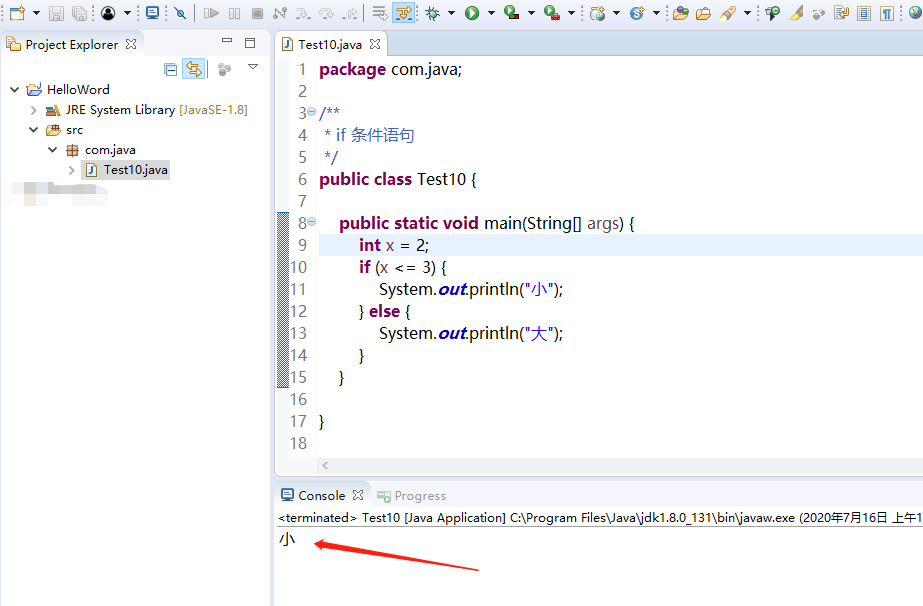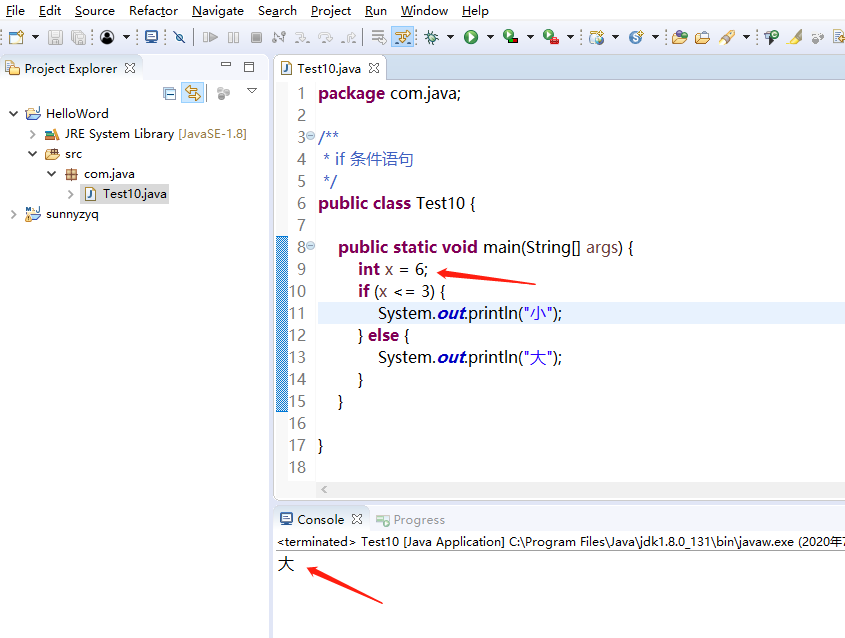int x = 10;
int y = (x < 10) ? 0 : 1;

int x = 10;
int y;
if (x < 10) {
y = 0;
} else {
y = 1;
}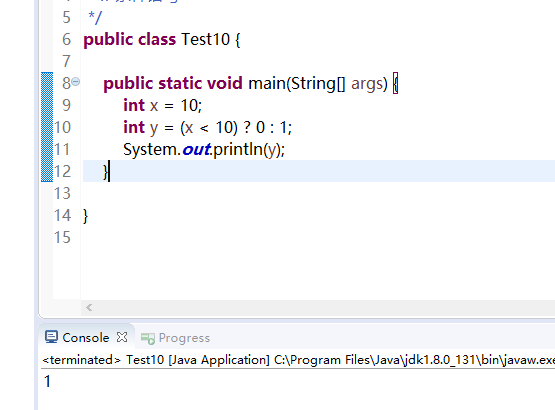if 运算结果：【3】if ... else if ... else...

if(点数为1或2) {
小
} else if(点数为3或4) {
中
} else {
大
}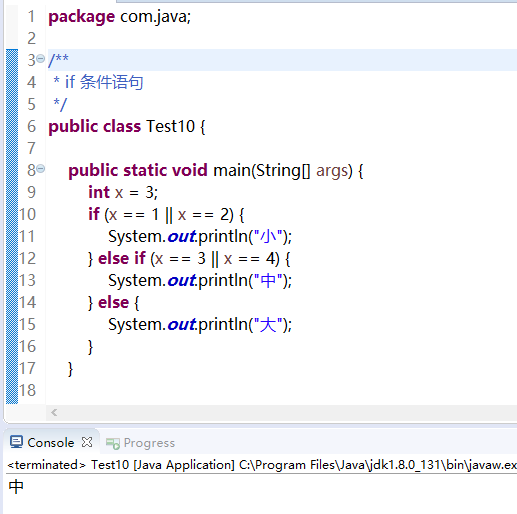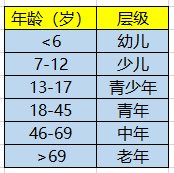if(age <6) {
幼儿
} else if(age >=7 && age <= 12) {
少儿
} else if(age >=13 && age <= 17) {
青少年
} else if(age >=18 && age <= 45) {
青年
}  else if(age >=46 && age <= 69) {
中年
} else {
大
}

【相关链接】

06-09495
03-30176411-04312
07-15203
06-132640
07-16223
12-15491
03-14323
05-1038
07-29615
©️2020 CSDN 皮肤主题: Age of Ai 设计师:meimeiellie